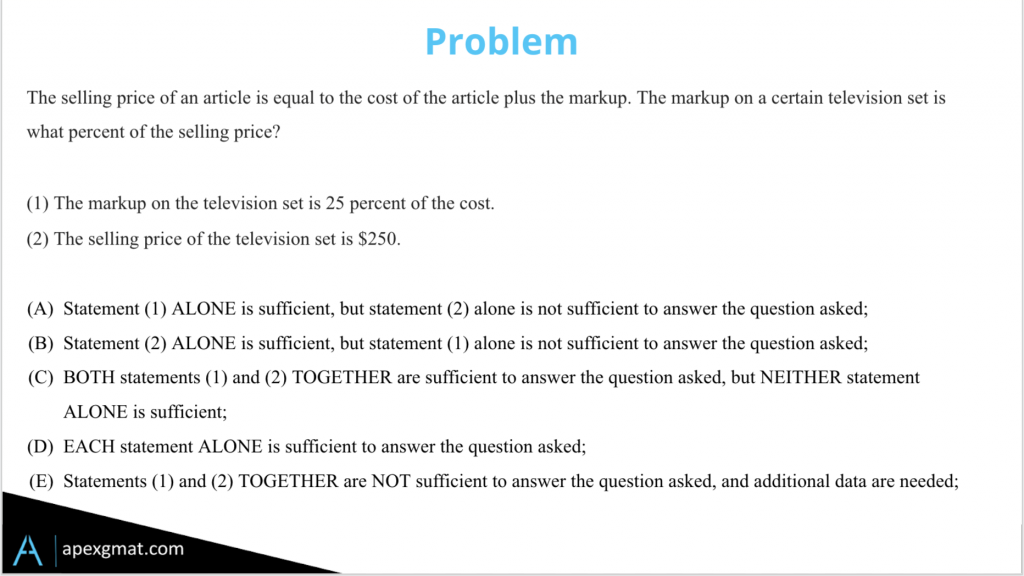Hey guys, today we’re going to take a look at a typically characteristic data sufficiency problem that gives us a relationship, and then asks us if we have enough to compute the final value of that relationship. There’s an algebraic solution path here, where they give us the equation and we need to see if we have all but one of the variables, that final variable being the one that they’re asking for. We can also do this via parts, scenario, and graphically, and we’ll take a look at all those as well.

###### GMAT Markup Problem IntroductionThis problem describes to us the relationship between the selling price, the cost, and the markup. And notice that, while we’re going to sketch it out here, the actual relationship doesn’t matter to us – all that matters is that if they’re asking for one term in terms of the rest if we have the other terms, that’ll be enough.

Algebraically we have selling price S equals the cost C plus the markup M. So this is giving us the markup in, let’s say dollar terms, whereas we might also set this up as selling price equals cost times one plus the markup percentage. And here we just have that notational shift. So, what we’re looking for, if we want to know the markup relative to the selling price, is an understanding of it either relative to the selling price or relative to the cost. That is, these two things are associated and the markup, when associated with the cost, gives us a ratio. Where the markup, when associated with the selling price, is a fraction. And if you’ll remember notationally these things are expressed differently, but conceptually there’s the same math behind it.

###### Statement 1

Number one gives us in percentage terms the mark up compared to the cost. So, here we can see it as 25% more and this is where it ties into that second version of the algebraic one we just looked at. The cost we can break up into four parts of 25% so that when we add the markup that’s a fifth part. Therefore, the markup comprises one-fifth or 20% of the selling price.

###### Statement 2

Number two provides us a concrete selling price but doesn’t tell us anything about the markup or the mix of cost versus markup as a percentage of the total selling price. Two is insufficient on its own, and as we’ve seen in many other data sufficiency problems, what they’re trying to do here is fool us into thinking we need a specific price, a discrete value to get sufficiency. When the question stem is asking us only for a relative value and when we’re being asked for a relative value, a percentage, a fraction, a ratio be on the lookout for fooling yourself into thinking that you need an anchor point a specific discrete value.

I hope this helps. If you enjoyed this GMAT Markup Problem, try your hand at this Triangle DS Problem.